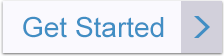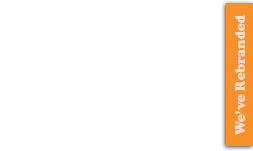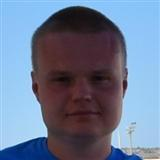Math_and_ITOnline
Tutor in:
Applied Mathematics
Languages:
English, Russian

Stuck with math? A tutor and mentor is here. I can provide assistance in many subjects of math: from algebra to calculus, from discrete mathematics to differential equations.

Degrees

I have a master's degree in Mathematics and Computer Science from the National University of Kyiv.

My Tutoring Service

I have deep knowledge and vast experience in all branches of mathematics, especially in Algebra and Trigonometry, Calculus (1,2 and 3), Discrete Mathematics, Statistics, Probability Theory, Differential Equations, Linear Algebra. I will explain everything in a high-quality manner.

Experience & Qualifications

I am a math tutor for more than 5 years. I teach students in various fields of mathematics. I can assist you with algebra, linear algebra, calculus, trigonometry, differential equations, and probability theory.